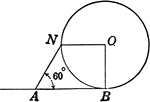Quadrant of Circle With 60 degree Arc

Illustration of circle with quadrant and 60 degree arc.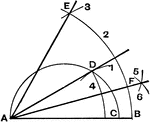Construct Equilateral Triangle

Method to construct an equilateral triangle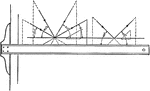Drawing Lines using Triangle, 30-, 45-, 60-Degrees

The arrows shows the motion direction of drawing a 30-degrees, 45-degrees, or a-60 degrees angle using…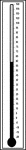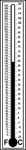Dual Lab Thermometers 60 Degrees Celsius Centigrade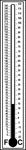Dual Lab Fahrenheit Thermometers

Dual Lab Thermometers 60 Degrees Fahrenheit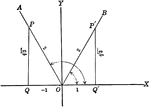Trigonometric Reference Triangles/Angles (60 degrees) Drawn in Quadrants

Trigonometric reference triangles/angles drawn for 60 degree reference angel in quadrants I and II.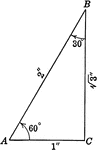Special Right Triangle with Angles 30, 60, 90 degrees

Special right triangle with angles 30 degrees, 60 degrees, and 90 degrees with side measures/relationships…